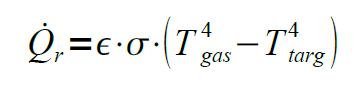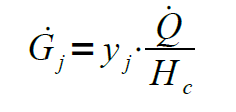# Prediction of Hazard

The simplest predictor for fire methods are the algebraic equations. Computer models are used to automate fire risk calculations and are particularly useful when many repeated calculations have to be made.

Once the design fire curve has been developed, it is then possible to predict the hazards that would result. The types of hazards that might be of interest include the following:

• Radiant heat flux, which affects the potential for ignition of materials and damage to structures or thermal injury to people.

• Smoke production, which dictates the volume of smoke produced.

• Fire plume and ceiling jet temperatures and velocities, which could cause weakening of exposed structural elements.

• Species production, which affects the rate at which an untenable environment could be created.

• Depth of upper layer, which can be used as a surrogate for an untenable environment.

The hazards that are quantified are a function of the objectives of the analysis. For example, if the purpose of the analysis is to determine if a thermally activated detection or suppression system is activated, only the temperatures and velocities of the boom and the ceiling jet can be determined.

The radiant heat flux is a measure of the radiative heat transfer rate per unit area. The radiant heat flux of a single element in combustion can be predicted as a function of the distance from the element. For radiant heat fluxes resulting from fire gases, as in a compartment fire, the radiant heat flow can be calculated if the gas temperature and the temperature of the target object are known by applying the following equation:where Q˙ r is the rate of radiant heat transfer (kW), e is the emissivity of gas (0 ≤ e ≤ 1), and s is the Stephan- Boltzmann constant (5.67 × 10–11 kW∙m−2·K−4), T gas is the temperature of gas (K) and T targ is the temperature of target (K).

## Fire Plumes and Ceiling Jet Temperatures and Velocities:

A fire will produce a plume of hot gas that will rise and contact the ceiling of a given space (for a compartment or enclosed space), forming a ceiling jet. Similarly, the temperature and velocity of a ceiling jet can be calculated.

## Species Production:

Fires can create a series of combustion products that can be toxic or corrosive, including carbon dioxide (CO2), water vapour (H2O), carbon monoxide (CO) and many others that vary with fuel and the combustion conditions. The production rates of the species can be calculated from the following equation,Where G˙ j is the smoke production rate of species j (kg∙s−1), yj is the yield fraction of species j, and ΔHc is the heat of combustion of fuel (kJ∙kg−1). Yield fractions for several fuels are available in the Society of Fire Protection Engineers (SFPE) “ Handbook of Fire Protection Engineering”.

## Depth of Upper Layer:

As smoke is produced in a compartment, it forms a layer that descends as a function of time. This is analogous to filling a container with water. However, it must be taken into account that the mass production rate of smoke is not constant, since as the layer falls, the rate of smoke production decreases due to the reduced vertical distance available to drag air towards the plume.

## Toxicity:

Toxic gases produced by a fire can incapacitate or kill people who are exposed to them. A commonly used approach to determine whether the environment induced by fire is potentially harmful to exposed people is the fractional effective dose (FED) model developed by the Purser. This can be expressed as follows:

where FINC is the fraction of an incapacitating dose of all asphyxiating gases, FCO is the fraction of an incapacitating dose of CO, FHCN is the fraction of an incapacitating dose of HCN, FIRR is the fraction of irritant dose, VHYP is the multiplication factor for carbon doixide (CO2) induced hyperventilation, FLOX is the fraction of an incapacitating dose of low-oxygen hypoxia, FIDC is the fraction of an incapacitating dose of CO2.